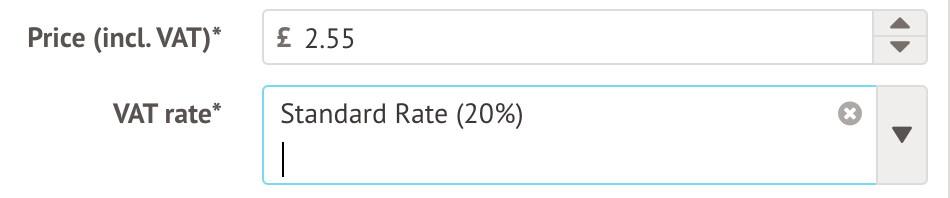# How is VAT calculated?

There are two scenarios where you may find the total amounts are different to your initial estimates after VAT is applied.

## For meal prices that include VAT

When setting up pricing for meals, you choose the price of the item including VAT as the total amount, and select what percentage of that is VAT.Arbor's invoicing system stores prices in the format of the ex-VAT amount and then the VAT rate. When storing these amounts, we use a HMRC-approved VAT rounding rule that states any fractions of a penny must be rounded up (if 0.5p or more) or down (if less than 0.5p).

So in this example, the price ex-VAT, would be 2.55 ÷ 1.20 = 2.125

This means when claiming back the VAT, the payment would be 2.55 - 2.125 = 0.425 which is 42.5 pence. It is not possible to pay back half a pence, so this is rounded up to the nearest penny.

This increases the total price including VAT up to £3.56.

## For payouts and reconciliation in bulk

In Arbor, VAT is charged per transaction fee as per our transaction fee policy, not calculated on the total amount for each weekly payout. This is in line with the VAT Traders’ Records Manual.

For each transaction, we round up to the nearest penny when the VAT comes to more than 0.5 of one penny. If lower, we round down. For example:

• If a £2 payment is processed through Arbor, the Stripe fee of 1.275% will be £0.02. VAT is then calculated on this £0.02, which would equate to £0.004 (less than half a penny) and therefore the VAT is rounded down to £0.00.
• If a £3 payment is processed through Arbor, the Stripe fee of 1.275% will be £0.03. VAT is then calculated on this £0.03, which would equate to £0.006 (over half a penny) and therefore VAT is rounded up to £0.01.